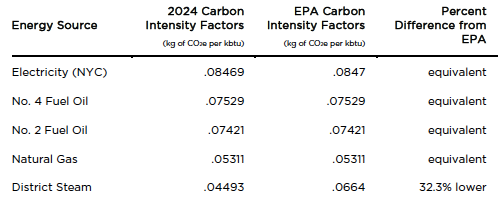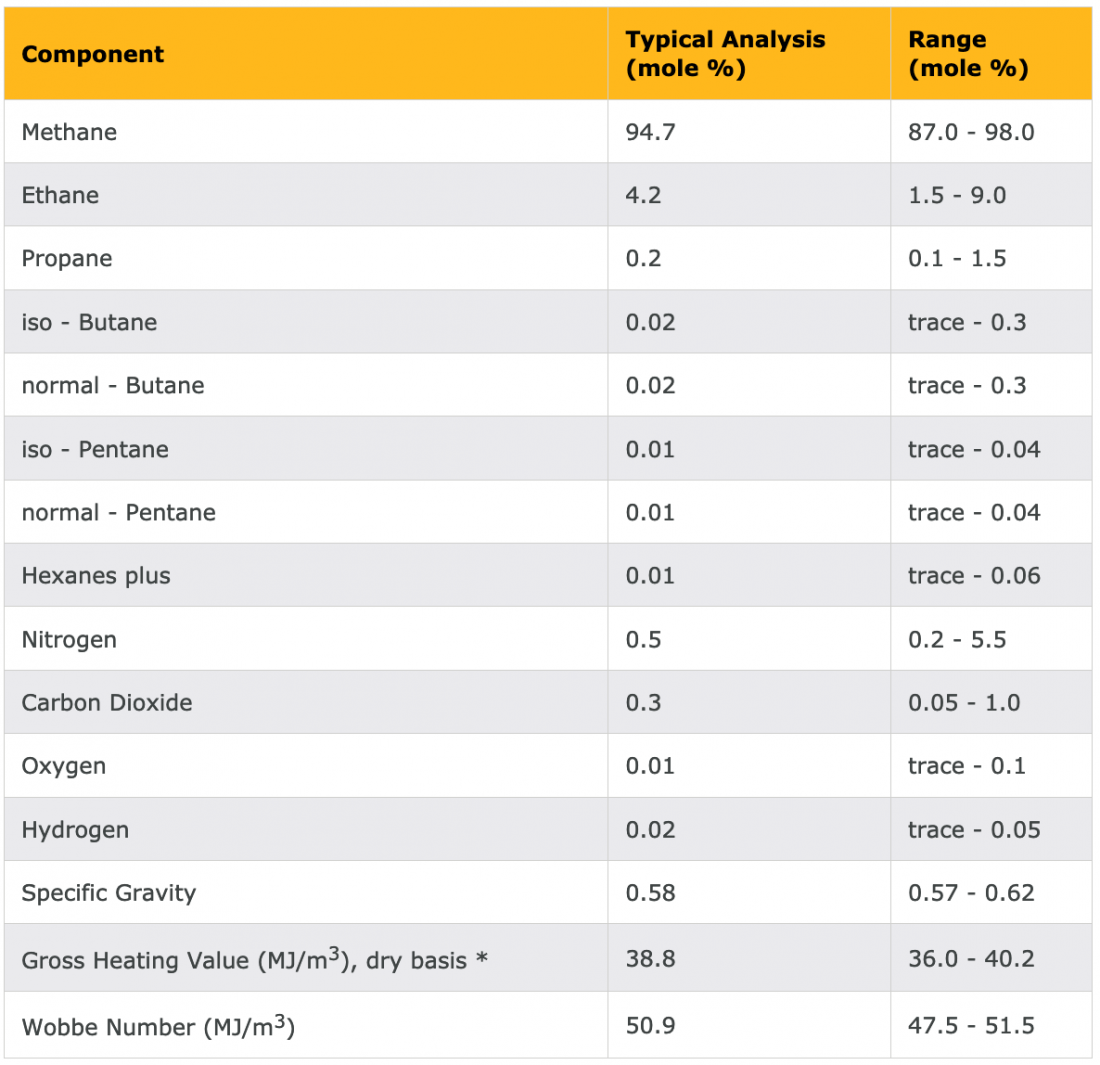# First-Principle Chemistry: Carbon Intensity

My last two blogs (May 4th and 11th) dealt with the challenges inherent in a new law that mandates carbon footprint reduction within large buildings in New York City, where I live and work. As with many other laws, there’s a penalty for noncompliance. The key question, of course, is: how do we know if we are complying? This quandary brings into doubt the efficacy of the law. According to the City, this problem is solved by a requirement that each report be signed by an energy expert, who can identify excessive levels and advise us on how to reduce our carbon emissions. Of course, since we are the ones who would have to pay the fine, it would be helpful if we knew how to find these numbers ourselves so that we could judge whether or not we need an energy professional in the first place—if it turns out we don’t, we need a better system.

Several years ago, the City passed a law that required large buildings to submit reports of their energy use. At the time, the penalty only applied if the building neglected to submit such a report. The energy report was supposed to provide the data from which the carbon emissions could be calculated, via carbon intensity factors—which I referred to in the last two blogs and am reposting again in Table 1. Once we have these data, the calculation is relatively simple: in your energy report, you state your energy sources and the quantity of energy that you used over a set period of time. You then multiply that quantity by the appropriate carbon intensity factor (as shown below) to find your total carbon emissions (in kg of carbon dioxide). Finally, you divide this number by the building’s square footage and check the result against the law’s limits.

The problem is that most of us have no idea where the numbers in Table 1 come from.

Table 1 – Carbon intensity factorsCarbon intensity factors originate in the chemical composition of the fuel. My job in this blog and next week’s follow up is to show the connection. Today, I’m looking at the relatively simple case of natural gas; next week’s blog will focus on the more complicated—but very important—case of electricity.

To quantify the connection between the chemical composition of a fuel and its carbon intensity, we need a bit of context in terms of the language of chemistry. When I teach these calculations to my students, many of them have no background in chemistry. I devote some time to the essence of the language. If you are challenged on this level but determined to learn more, you can “hire” your high-school kid, or if needed, the kid of your neighbor (properly compensated) who is taking or has taken a chemistry class to be your chemistry Tsar. Table 2 shows a typical chemical composition of natural gas.

Table 2 – Example of the chemical composition of natural gas* The gross heating value is the total heat obtained by complete combustion at constant pressure of a unit volume of gas in air, including the heat released by condensing the water vapour in the combustion products (gas, air, and combustion products taken at standard temperature and pressure).

As we can see in Table 2, natural gas is almost pure methane (CH4). For simplicity’s sake, I will assume here that it is pure methane. Next week, I will delve into how to handle the traces of other chemicals that show up in such tables.

The calculation, shown below, describes the simple chemical reaction of burning methane (reacting it with oxygen—O2). I also include the energy conversion from Btu to Calories:

CH4 + 2O2 → CO2 + 2H2O + 210 Cal

1 Btu = 0.252 Cal

Converting kbtu to Cal:
1 kbtu = 1000 Btu
1 kbtu = (1,000 Btu) * 0.252 Cal/Btu = 252 Cal

One more definition that might be useful here is that of a mole. According to Encyclopedia-Britannica:

Mole, also spelled mol, in chemistry, [is] a standard scientific unit for measuring large quantities of very small entities such as atoms, molecules, or other specified particles.

The concept lets us calculate the interactions between molecules, expressed in chemical reactions, and macroscopic quantities, measured in grams.

Back to the chemical equation:

Burning 16g (1 mole) of methane (CH4) produces 44g (1 mole) of carbon dioxide (CO2) + 36g of water (2 moles of H2O) + 210 Cal of energy.

That means that production of 210 Cal from burning methane is associated with 0.044 kg of carbon dioxide emissions. So, the carbon intensity of methane is:

0.044kg CO2/210 Cal = 0.0002096 kg/Cal = 2.1*10-4 kg CO2/Cal
= (2.1*10-4 kgCO2/Cal)*(252 Cal/kbtu) = 0.0529kg CO2/kbtu

That’s identical to the value in Table 1.

Unfortunately, most gas bills in the US use units of therms. Among all physical indicators, energy is the one that is expressed with the largest variety of units (mostly for historical reasons). Therefore, when we are trying to quantify energy use, one of the most demanding tasks is to correctly apply the needed energy conversions.

In the case of natural gas, the approximate conversions are:

1 therm = 100,000 Btu = 25,000 Cal = 100 SCF (Standard Cubic Feet)

SCF is often used to express the quantity of material—your gas bill reflects both the amount of energy (therms) and the volume of gas you are using (SCF).